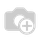# UML Diagrams for OpenERP

Hi,

Can anyone help me with the UML (Unified Modeling Language) diagrams for OpenERP?

I am developing one of the ERP as my university project for 'Real Estate Builder's Perspectives'.

Also want to draw 'Entity-Relationship diagram'(E-R diagram) and 'Data Flow diagram'(DFD diagram)

UML diagrams:

1. Class diagram

2. Use Case diagram

3. Activity diagram

4. Sequence diagram

5. Component diagram

6. Deployment diagram

7. Object diagram

8. Collaboration diagram

9. State Chart diagram